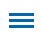Solving single-step or multistep practical mathematical problems is a theme throughout the Common Core Standards and Virginia's Standards of Learning. These practical problems require computation skills that evolve as the students progress from kindergarten to high school. Although this authentic task can be utilized by students in multiple grades, for fourth graders, the problems typically involve adding, subtracting, and multiplication as well as division with whole numbers.

The On Top of Denali template involves a problem solving strategy that utilizes subtraction as a potential part of a Computation unit taught at the start of the school year. Students are asked to compare the elevation of Denali to the highest points of other states including Oregon, Arkansas, and Virginia to name a few.

Instructional Concept: Solving practical problems by subtraction whole numbers through the ten thousands.Location: Templates > Math > Numbers > Computation

Estimated Completion Time: 15-20 minutes

Common Core State Standards for Mathematics

CCSS.MATH.CONTENT.4.OA.A.3
Solve multistep word problems posed with whole numbers and having whole-number answers using the four operations, including problems in which remainders must be interpreted. Represent these problems using equations with a letter standing for the unknown quantity. Assess the reasonableness of answers using mental computation and estimation strategies including rounding.

Virginia Standards of Learning - Mathematics

SOL.M.4.4.D
The student will create and solve single-step and multistep practical problems involving addition, subtraction, and multiplication, and single step practical problems involving division with whole numbers.

Editor's Note: This blog is the sixth in a series of posts from former math specialist Scott Loomis. In these posts, he shares more about the real world math templates he has created for Wixie and outlines how you can use and modify them for your own awesome performance tasks. You can read the backstory on his experience and expertise below.